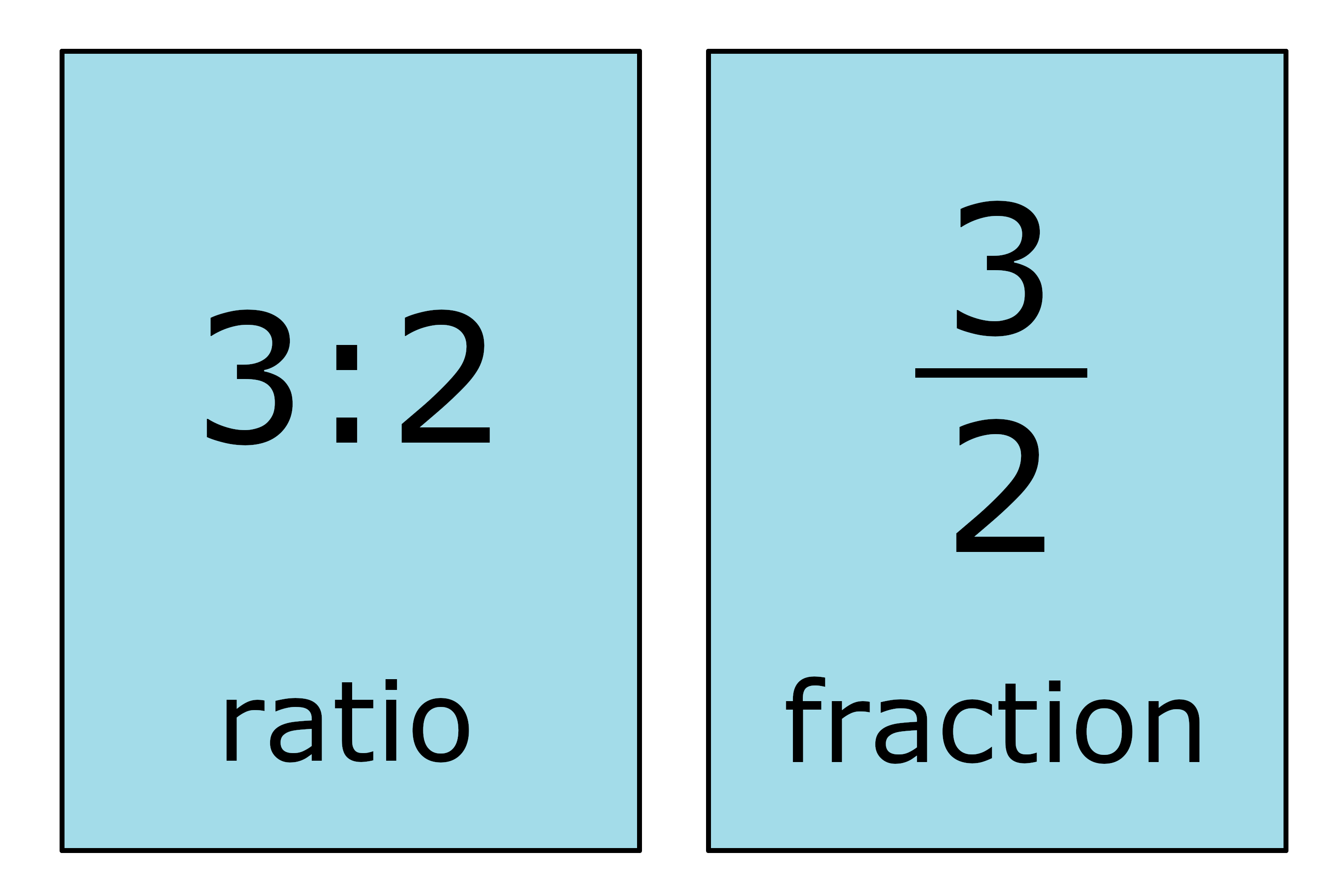# Ratio to Fraction Calculator

Convert a ratio to a fraction by entering both parts of the ratio below.

Ratio
:

## Solution:

### Part to Whole Fraction

2 / 3

#### Steps to Solve a Part to Whole Fraction

Step One: Rewrite the ratio as a fraction
4 : 6=4 / 6
Step Two: Reduce and simplify the fraction
4 / 6=2 / 3

### Part to Part Fractions

2 / 5  &  3 / 5

#### Steps to Solve a Part to Part Fraction

Step One: Set the denominator as the ratio's left side + the right side
denominator=4+6=10
Step Two: Set the left side and right side of the ratio over the denominator
4 / 10&6 / 10
Step Three: Reduce and simplify each fraction
4 / 10=2 / 5
6 / 10=3 / 5
Learn how we calculated this below

## How to Convert a Ratio to a Fraction

A ratio can be expressed as a fraction in two different ways. A ratio can be a part-to-whole or a part-to-part ratio. The methods for converting a ratio to each type of fraction are a little different.

The calculator above will find both. Continue reading to learn how to convert a ratio to a fraction.

### How to Convert a Part-to-Whole Ratio to a Fraction

A part-to-whole ratio is an expression of the relationship between a subset and the entire set. For example, the proportion of peaches to a basket of fruit can be expressed as a part-to-whole ratio.

To convert a part-to-whole ratio to a fraction, simply rewrite the ratio as a fraction. The left side of the ratio is the numerator, and the right side is the denominator.

The fraction can optionally be reduced after converting if needed.

For example, let’s convert 3:2 to a fraction.

3:2 = 3 / 2### How to Convert a Part-to-Part Ratio to a Fraction

A part-to-part ratio is an expression of the relationship between two subsets of a set. For example, the proportion of peaches to oranges in a basket of only peaches and oranges can be expressed using a part-to-part ratio.

A part-to-part ratio is converted to two fractions. Each fraction is equivalent to a part-to-whole ratio for each of the subsets.

To convert, start by adding both parts of the ratio together. This will be the denominator for each of the fractions and also represents the whole set.

Then, each part of the ratio creates a fraction, with the numerators being the first and second parts of the ratio and the denominator being the sum that was just found.

The result will be two fractions that represent the proportion of the subset to the whole set.

For example, let’s convert the ratio of 1 peach to 3 oranges in a fruit basket, or 1:3, to fractions.

First, find the denominator.

denominator = 1 + 3
denominator = 4

Then, put each part of the ratio over the denominator to find the fractions.

peaches = 1 / 4
oranges = 3 / 4

Thus, 1 / 4 of the fruit in the basket are peaches, and 3 / 4 of the fruit in the basket are oranges.

You might also find our fraction to ratio calculator useful.